## Saturday, May 14, 2011

### Graphing pitch and volume

Different ways of displaying pitch and (measured a little later) volume using TurtleArtwith only dots at the end of the line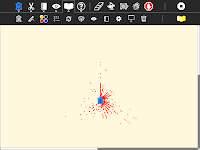colour determined by volume (measured a little later again)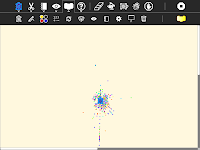x = pitch, y=volume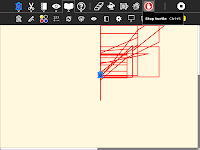Challenges:
• No code is given here, create these graphs
• Make other graphs
• A third variable to graph is number of occurrences of a pitch/volume pair
• Can you get useful information from these graphs? Can you tell speech from music, TV program from advertisment?
• The pitch block only shows the pitch of the loudest component. This is derived from a FFT (Fast Fourier Transform). Can you program a Python block to show all the spectral components and their amplitude? (Look at the source to see how the FFT is done)
• With more than one laptop you can log more data. Can you combine data from multiple laptops and graph it? How will you synchronize the data?

Labels: , , ,

## Monday, May 09, 2011

### Turtle Art and USB serial devices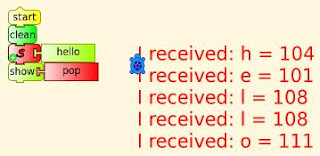Using the Python block to interface with a USB serial device (tested with the Arduino)

Requires the Pyserial library. The recommended install procedure does not work because it requires a developer installation of Python python2.6-dev

Instead copy the serial directory here to the directory home/olpc/Activities/TurtleArt.activity

Load the Python block with this code

Tested on an Arduino, the Arduino had a simple echo program loaded using the Arduino IDE on another computer but this should work with any board with a FTDI FT232R USB serial chip

Ideas for controlling devices with the FTDI FT232R USB serial chip here

Labels: , , , ,

## Sunday, May 08, 2011

### Turtle calculus

Its easy to plug in various functions as a graph's gradient in Turtle Art. The resulting graph is the integral of the function used as the gradient. In the below case, the gradient is 1 and its integral is a straight line, f(x)=x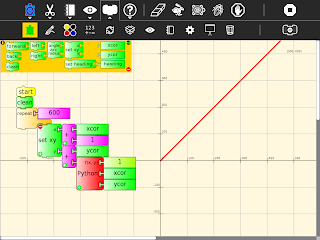Plug in a linear gradient, -2+x/100, and make a parabola with zero crossings at x=0 and x=400A quadratic gradient generates a cubic with zero crossings at x=0 and x=300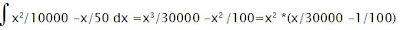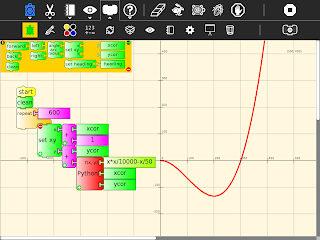The integral of sin is -cos(by the chain rule)making dy/dx =y gives an exponential curve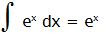but a nonzero value at x=0 is necessary, in this case forward 1 means f(0)=1
starting at 0, f(0)=0 has a solution of a straight line f(x)=0Challenges
Graph for negative x
Graph the exponential for f(0)=-1 explain
The exponential above is for dy/dx = y/100 what exactly is the formula for the graph?
Find more interesting integrals
Graph the derivative of a function
Iteratively solve d2y/dx2 =-y
Explain the offset in the graph of -cos
A step size of 1 is used, what error does the step size introduce?

Labels: , , , , ,